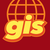# Python Extent Calculations

1541
5
08-19-2021 05:24 AMNew Contributor III

Good Morning,

Does anyone know if it is possible to field calculate the extent of a polygon in a projection other than the projection the feature class resides in.

This python field calculate code Expression: !shape.extent.XMax! works perfect for the feature classes current projection, but I cannot find any reference to projecting that extent value differently.

We are currently in a state plane projection (WKID 2253) and would like to have the field values in 4326 (decimal degrees) to pull those field values into other non GIS software.

You can do this with point centroid calculations, but the same concept does to apply to extents.

'arcpy.PointGeometry(!Shape!.centroid,!Shape!.spatialReference).projectAs(arcpy.SpatialReference(4326)).centroid.Y'

Yes I know I can use add geometry attributes to do what I would like, but because that tool adds four new fields to a new feature class, I would prefer not to back join and field calculate to fill in the fields needed.

Tags (3)
1 Solution

Accepted SolutionsbyMVP Frequent Contributor

Code Block:

``````def get_projected_extent(shape, wkid):
in_sr = shape.spatialReference
out_sr = arcpy.SpatialReference(wkid)
# get the extent
e = shape.extent
# convert the extent into a geometry
# either as polygon of all 4 points or as a diagonal polyline
e_points = [
arcpy.Point(e.XMin, e.YMin),
arcpy.Point(e.XMax, e.YMax),
]
e_geometry = arcpy.Polyline(arcpy.Array(e_points), in_sr)
# project the geometry and get the projected extent
e_proj = e_geometry.projectAs(out_sr).extent
return e_proj``````

Field =

``get_projected_extent(!SHAPE!, 4326).XMax``

Have a great day!
Johannes
5 RepliesNew Contributor III

in python 3. x. install pyproj (from arcgis pro) or directement from https://pyproj4.github.io/pyproj/stable.

get xy from shape and convert to any other  coordinates.

example:

from pyproj import CRS
from pyproj import Transformer

lon = 166.450307
lat = -22.307108
sr_in = CRS("EPSG:4326"
sr_out = CRS("EPSG:3857")
mytransformationTransformer.from_crs(sr_insr_out)
xy = transformer_Caledonie.transform(latlon)byMVP Frequent Contributor

Code Block:

``````def get_projected_extent(shape, wkid):
in_sr = shape.spatialReference
out_sr = arcpy.SpatialReference(wkid)
# get the extent
e = shape.extent
# convert the extent into a geometry
# either as polygon of all 4 points or as a diagonal polyline
e_points = [
arcpy.Point(e.XMin, e.YMin),
arcpy.Point(e.XMax, e.YMax),
]
e_geometry = arcpy.Polyline(arcpy.Array(e_points), in_sr)
# project the geometry and get the projected extent
e_proj = e_geometry.projectAs(out_sr).extent
return e_proj``````

Field =

``get_projected_extent(!SHAPE!, 4326).XMax``

Have a great day!
JohannesNew Contributor III

This solution was successful with a caveat. You have to use the arcpy.polyline array with a diagonal polyline to get the extent and not the polygon array functionality. The polygon array runs without error but the field is not calculated.byMVP Esteemed Contributor

Not sure what you mean by "back join" since you can specify the coordinate system you want the extent values calculated in directly

Add Geometry Attributes (Data Management)—ArcGIS Pro | Documentation

So if you want, you can run the tool and get the values calculated in geographic coordinates, then run again to get them calculated in a projected coordinate system by just changing the desired coordinate system. The downside is that the field values are overwritten between runs, which is annoying if you want both geographic and projected coordinates for the extent values in the same table.

Alter field Alter Field (Data Management)—ArcGIS Pro | Documentation could be used to rename the derived fields to differentiate between the gcs and pcs values, but annoyingly, you have to run this for each field.... they need an "Alter FieldS" tool.

... sort of retired...New Contributor III

I don't want a new output, although those tools work. I want the values calculated in an existing field.